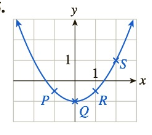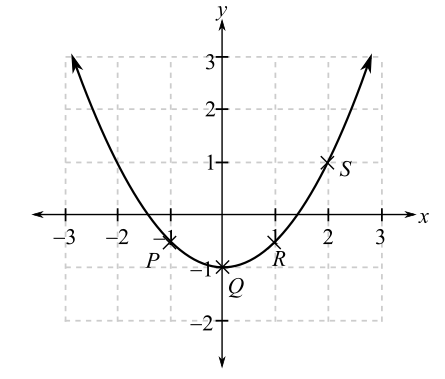Chapter 10, Problem 35REFinite Mathematics and Applied Cal...

7th Edition
Stefan Waner + 1 other
ISBN: 9781337274203

Solutions

Chapter
SectionFinite Mathematics and Applied Cal...

7th Edition
Stefan Waner + 1 other
ISBN: 9781337274203
Textbook Problem

In Exercise 35-38 you given the graph of a function with four points marked. Determine at which (if any) of these points the derivate of the function is (a)-1, (b) 0, (c) 1, and (d) 2.(a)

To determine

The labelled points on the graph that has value of the slope of tangent is 1. The provided graph is:Explanation

Given information:

The provided graph is:

When function is increasing or rising the slope of the function is positive and greatest where the tangent is more slanted whereas when the function is decreasing or falling its slope is negative and it has the least value where the tangent is the most slanted...

(b)

To determine

The labelled points on the graph that has value of the slope of tangent is 0.

The provided graph is:(c)

To determine

The labelled points on the graph that has value of the slope of tangent is 1.

The provided graph is:(d)

To determine

The labelled points on the graph that has value of the slope of tangent is 2. The provided graph is:Still sussing out bartleby?

Check out a sample textbook solution.

See a sample solution

The Solution to Your Study Problems

Bartleby provides explanations to thousands of textbook problems written by our experts, many with advanced degrees!

Get Started

Polynomials Find the sum, difference, or product. 15. (12x 7) (5x 12)

Precalculus: Mathematics for Calculus (Standalone Book)

A sample of n = 9 scores has X = 108. What is the sample mean?

Essentials of Statistics for The Behavioral Sciences (MindTap Course List)

In Exercises 4144, determine whether the statement is true or false. 42. 5 5

Applied Calculus for the Managerial, Life, and Social Sciences: A Brief Approach

In Problems 1-6 simplify each fraction. 6.

Mathematical Applications for the Management, Life, and Social Sciences

Continuity of a Function Discuss the continuity of the function h(x)=x[x].

Calculus: Early Transcendental Functions (MindTap Course List)

True or false:

Study Guide for Stewart's Multivariable Calculus, 8th

In Exercises 2532, find the inverse of the matrix if it exist. A=

Finite Mathematics for the Managerial, Life, and Social Sciences